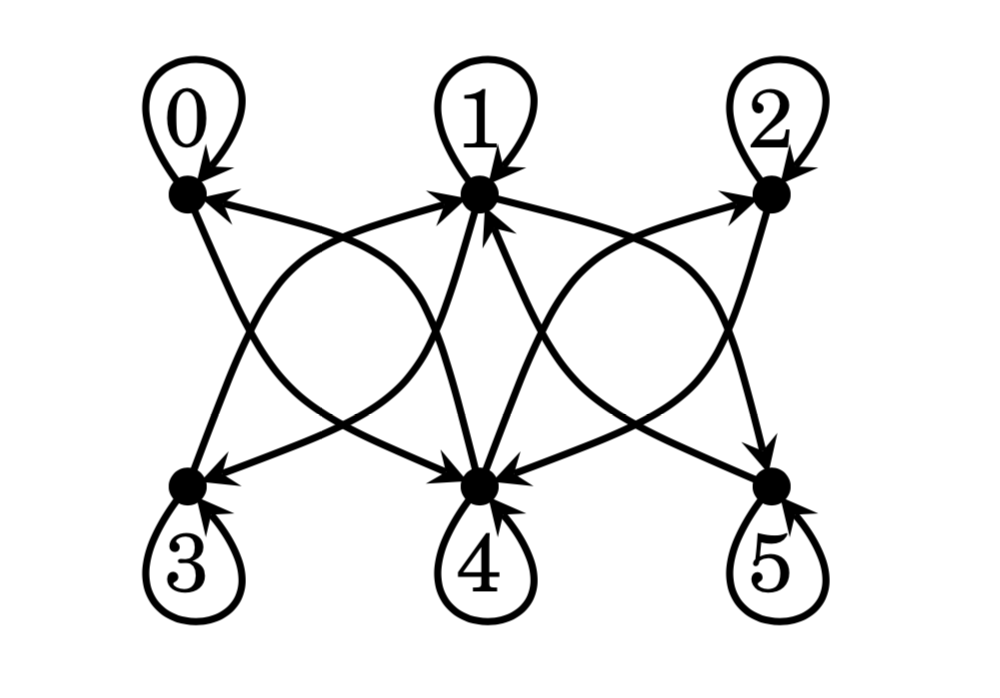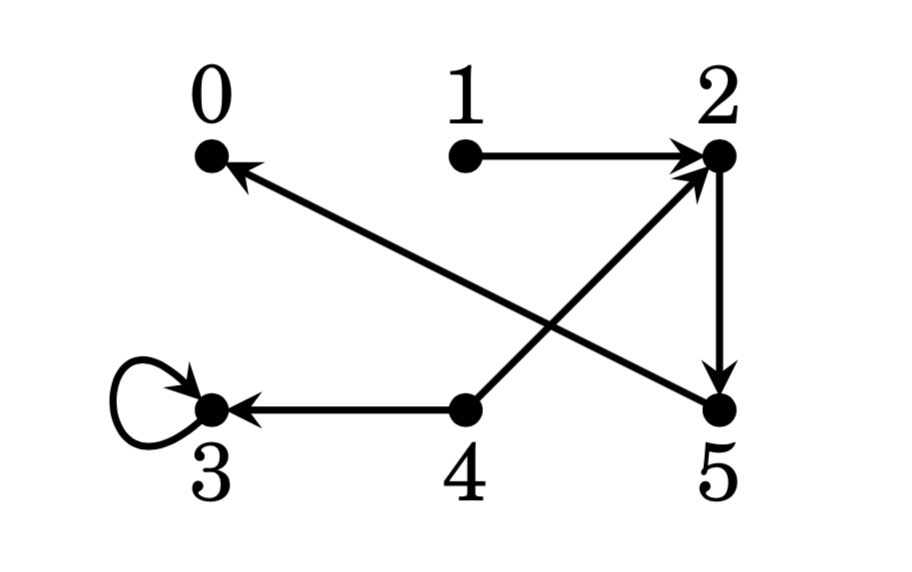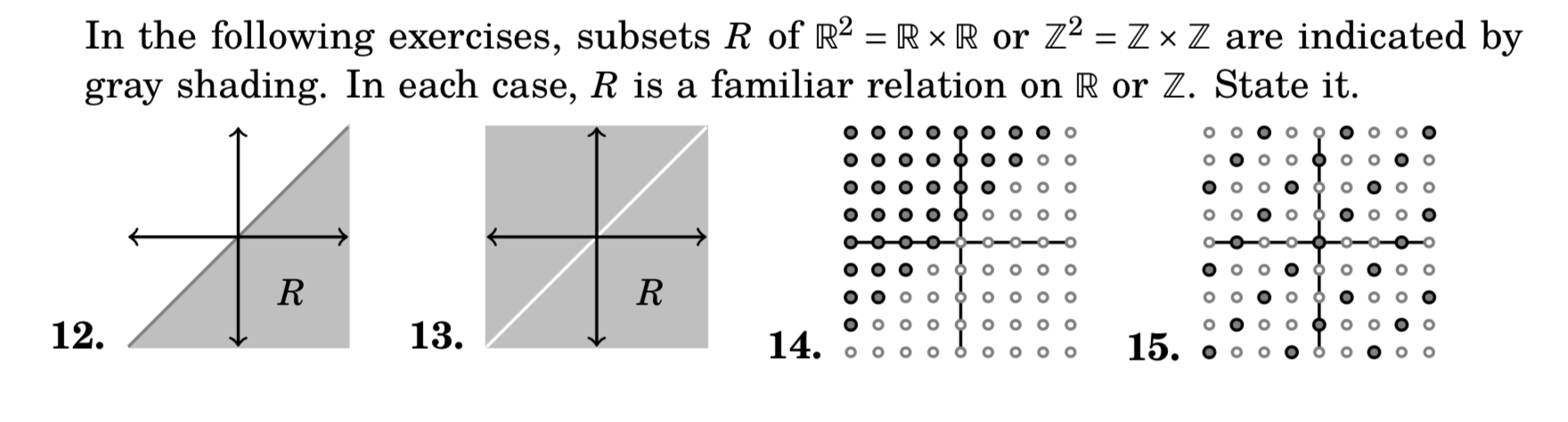## Relations

The following is the list of problems for sections 11.0 and 11.1 of the Book of Proof (pages 178 and 182). There is a forum open at the end, so you can ask questions. It is a great way to interact with the instructor and with other students in your class, should you need some assistance with any question. Please, do not post solutions.

### Exercises for Section 11.0

1. Let $$A = \{ 0, 1, 2, 3, 4, 5\}.$$ Write out the relation $$R$$ that expresses $$>$$ on $$A.$$ Then illustrate it with a diagram.
2. Let $$A = \{ 1, 2, 3, 4, 5, 6 \}.$$ Write out the relation $$R$$ that expresses $$\vert$$ (divides) on $$A.$$ Then illustrate it with a diagram.
3. Let $$A = \{ 0, 1, 2, 3, 4, 5 \}.$$ Write out the relation $$R$$ that expresses $$\geq$$ on $$A.$$ Then illustrate it with a diagram.
4. Here is a diagram for a relation $$R$$ on a set $$A.$$ Write the sets $$A$$ and $$R.$$5. Here is a diagram for a relation $$R$$ on a set $$A.$$ Write the sets $$A$$ and $$R.$$6. Congruence modulo 5 is a relation on the set $$A = \mathbb{Z}.$$ In this relation $$xRy$$ means $$x \equiv y \pmod{5}.$$ Write out the set $$R$$ in set-builder notation.
7. Write the relation $$<$$ on the set $$A = \mathbb{Z}$$ as a subset $$R$$ of $$\mathbb{Z} \times \mathbb{Z}.$$ This is an infinite set, so you will have to use set-builder notation.
8. Let $$A = \{ 1, 2, 3, 4, 5, 6\}.$$ Observe that $$\emptyset \subseteq A \times A,$$ so $$R = \emptyset$$ is a relation on $$A.$$ Draw a diagram of this relation.
9. Let $$A = \{ 1, 2, 3, 4, 5, 6\}.$$ How many different relations are there on the set $$A$$?
10. Consider the subset $$R = ( \mathbb{R} \times \mathbb{R} ) \setminus \{ (x,x) : x \in \mathbb{R} \} \subseteq \mathbb{R} \times \mathbb{R}.$$ What familiar relation on $$\mathbb{R}$$ is this?
11. Given a finite set $$A,$$ how many different relations are there on $$A$$?### Exercises for Section 11.1

1. Consider the relation $$R = \{ (a,a), (b,b), (c,c), (d,d), (a,b), (b,a) \}$$ on set $$A = \{ a, b, c, d \}.$$ Is $$R$$ reflexive? Symmetric? Transitive? If a property does not hold, say why.
2. Consider the relation $$R = \{ (a,b), (a,c), (c,c), (b,b), (c,b), (b,c) \}$$ on set $$A = \{ a, b, c \}.$$ Is $$R$$ reflexive? Symmetric? Transitive? If a property does not hold, say why.
3. Consider the relation $$R = \{ (a,b), (a,c), (c,b), (b,c) \}$$ on set $$A = \{ a, b, c \}.$$ Is $$R$$ reflexive? Symmetric? Transitive? If a property does not hold, say why.
4. Let $$A = \{ a, b, c, d \}.$$ Suppose $$R$$ is the relation \begin{align} R = \{ &(a,a), (b,b), (c,c), (d,d), (a,b), (b,a), (a,c), (c,a), \cr &(a,d), (d,a), (b,c), (c,b), (d,b), (d,b), (c,d), (d,c) \}. \end{align}

Is $$R$$ reflexive? Symmetric? Transitive? If a property does not hold, say why.

5. Consider the relation $$R = \{ (0,0), (\sqrt{2},0), (0,\sqrt{2}), (\sqrt{2}, \sqrt{2}) \}$$ on $$\mathbb{R}.$$ Is $$R$$ reflexive? Symmetric? Transitive? If a property does not hold, say why.
6. Consider the relation $$R = \{ (x,x) : x \in \mathbb{Z} \}$$ on $$\mathbb{Z}.$$ Is $$R$$ reflexive? Symmetric? Transitive? If a property does not hold, say why. What familiar relation is this?
7. There are 16 possible different relations on the set $$A = \{ a, b \}.$$ Describe all of them. (A picture of each will suffice, but don’t forget to label the nodes.) Which ones are reflexive? Symmetric? Transitive?
8. Define a relation on $$\mathbb{Z}$$ as $$x\operatorname{R}y$$ if $$\lvert x-y \rvert < 1.$$
Is $$R$$ reflexive? Symmetric? Transitive? If a property does not hold, say why. What familiar relation is this?
9. Define a relation on $$\mathbb{Z}$$ by declaring $$x \operatorname{R} y$$ if and only if $$x$$ and $$y$$ have the same parity. Is $$R$$ reflexive? Symmetric? Transitive? If a property does not hold, say why. What familiar relation is this?
10. Suppose $$A \neq \emptyset.$$ Since $$\emptyset \subseteq A \times A,$$ the set $$R = \emptyset$$ is a relation on $$A.$$ Is $$R$$ reflexive? Symmetric? Transitive? If a property does not hold, say why.
11. Suppose $$A = \{ a, b, c, d \}$$ and $$R = \{ (a,a), (b,b), (c,c), (d,d) \}.$$ Is $$R$$ reflexive? Symmetric? Transitive? If a property does not hold, say why.
12. Prove that the relation $$\vert$$ (divides) on the set $$\mathbb{Z}$$ is reflexive and transitive. (Use example 11.8 as a guide if you are unsure on how to proceed.)
13. Consider the relation $$R = \{ (x,y) \in \mathbb{R} \times \mathbb{R} : x - y \in \mathbb{Z} \}$$ on $$\mathbb{R}.$$ Prove that this relation is reflexive, transitive and symmetric.
14. Suppose $$R$$ is a symmetric and transitive relation on a set $$A,$$ and there is an element $$a \in A$$ for which $$a \operatorname{R} x$$ for every $$x \in A.$$ Prove that $$R$$ is reflexive.
15. Prove or disprove: If a relation is symmetric and transitive, then it is also reflexive.
16. Define a relation $$R$$ on $$\mathbb{Z}$$ by declaring that $$x \operatorname{R} y$$ if and only if $$x^2 \equiv y^2 \pmod{4}.$$ Prove that $$R$$ is reflexive, symmetric and transitive.
17. Modifying the above exercise 8 slightly, define a relation $$R$$ on $$\mathbb{Z}$$ as $$x \operatorname{R} y$$ if and only if $$\lvert x-y \rvert \leq 1.$$ Say whether $$R$$ is reflexive. Is it symmetric? Transitive?
18. The table on page 179 shows that relations on $$\mathbb{Z}$$ may obey various combinations of the reflexive, symmetric and transitive properties. In all, there are $$2^3 = 8$$ possible combinations, and the table shows 5 of them. (There is some redundancy, as $$\leq$$ and $$\vert$$ have the same type.) Complete the table by finding examples of relations on $$\mathbb{Z}$$ for the three missing combinations.